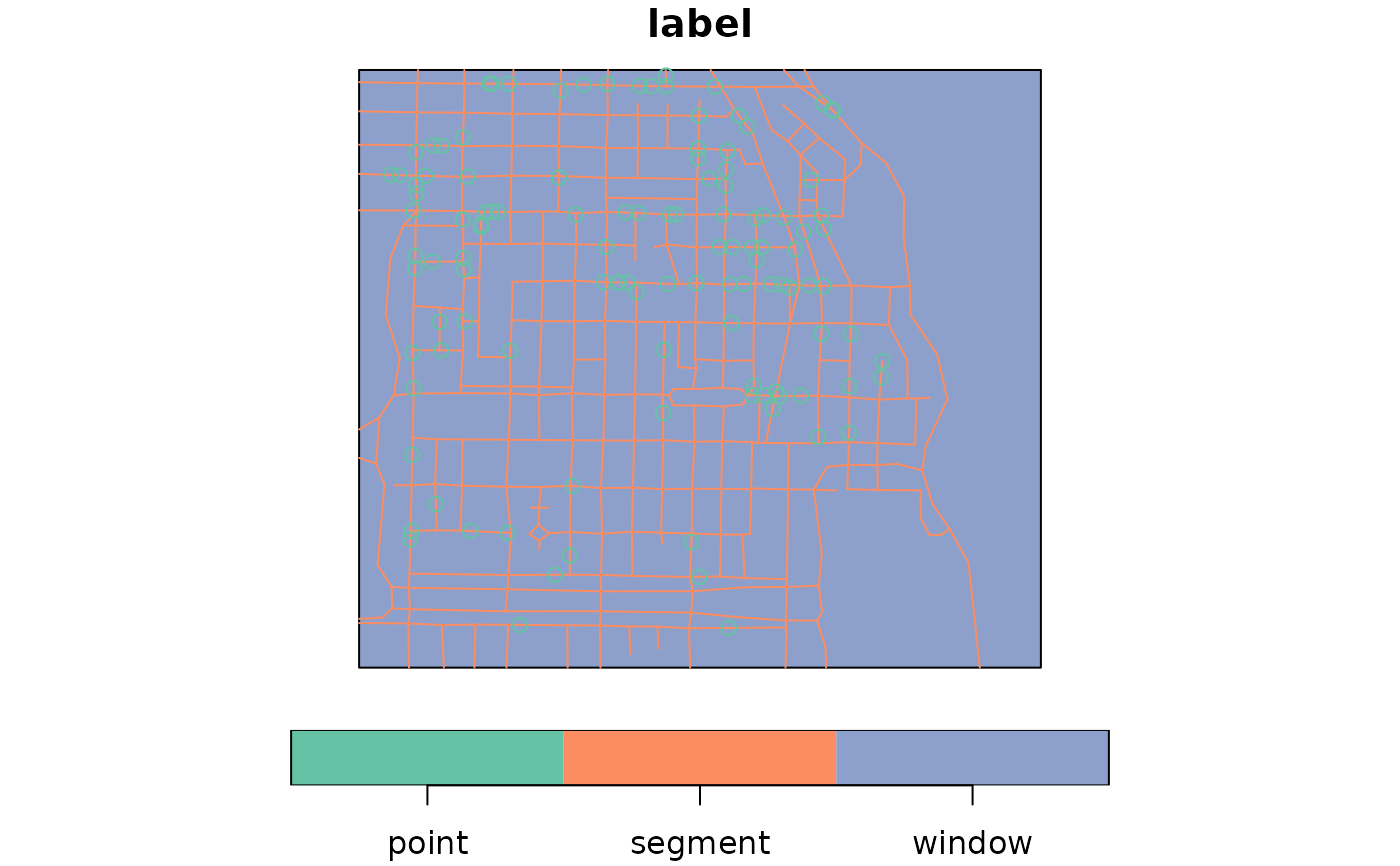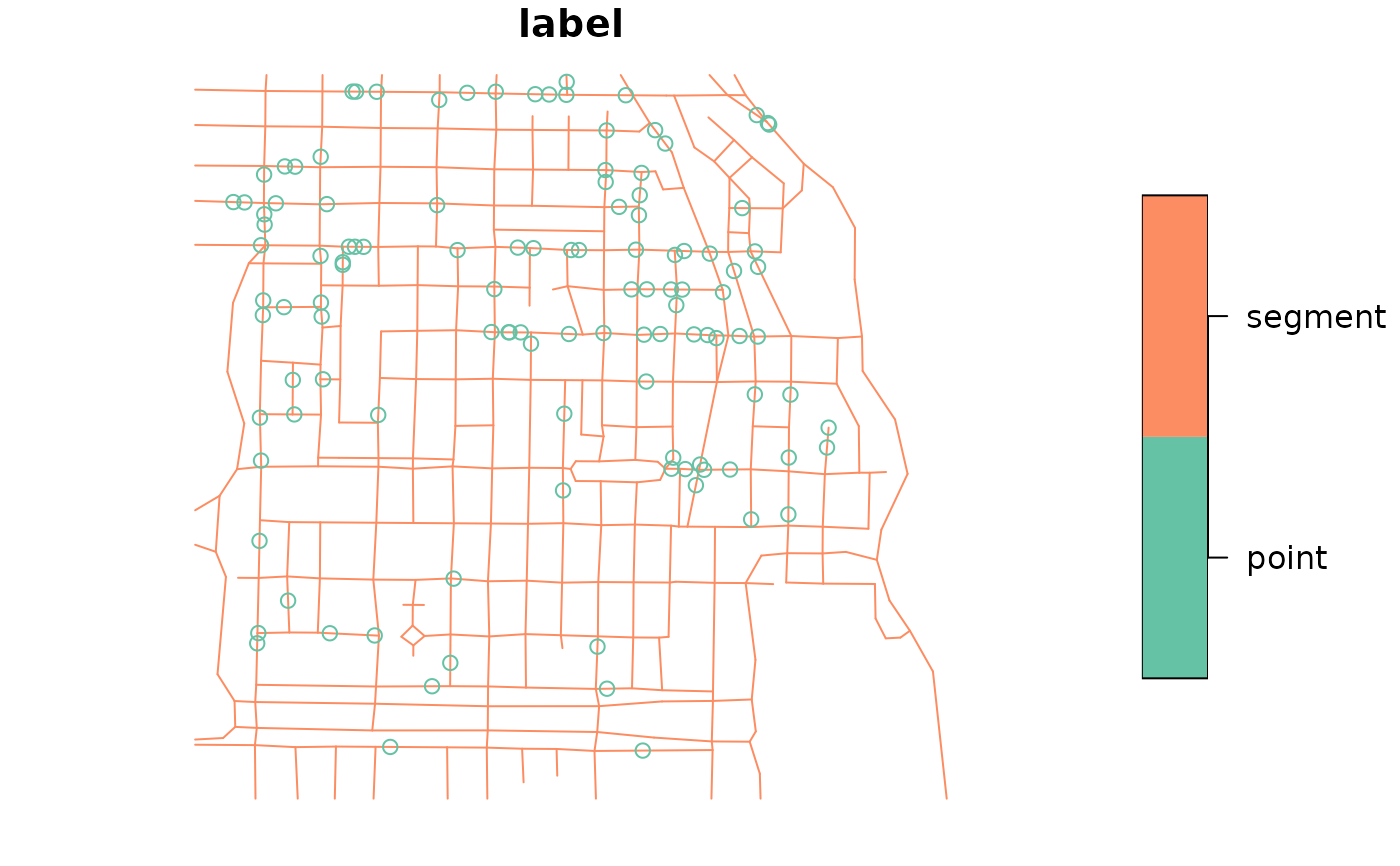Convert foreign object to an sf object

st_as_sf(x, ...)

# S3 method for data.frame
st_as_sf(
x,
...,
agr = NA_agr_,
coords,
wkt,
dim = "XYZ",
remove = TRUE,
na.fail = TRUE,
sf_column_name = NULL
)

# S3 method for sf
st_as_sf(x, ...)

# S3 method for sfc
st_as_sf(x, ...)

# S3 method for Spatial
st_as_sf(x, ...)

# S3 method for map
st_as_sf(x, ..., fill = TRUE, group = TRUE)

# S3 method for ppp
st_as_sf(x, ...)

# S3 method for psp
st_as_sf(x, ...)

# S3 method for lpp
st_as_sf(x, ...)

# S3 method for s2_geography
st_as_sf(x, ..., crs = st_crs(4326))

## Arguments

x

object to be converted into an object class sf

...

passed on to st_sf, might included named arguments crs or precision

agr

character vector; see details section of st_sf

coords

in case of point data: names or numbers of the numeric columns holding coordinates

wkt

name or number of the character column that holds WKT encoded geometries

dim

passed on to st_point (only when argument coords is given)

remove

logical; when coords or wkt is given, remove these columns from data.frame?

na.fail

logical; if TRUE, raise an error if coordinates contain missing values

sf_column_name

character; name of the active list-column with simple feature geometries; in case there is more than one and sf_column_name is NULL, the first one is taken.

fill

logical; the value for fill that was used in the call to map.

group

logical; if TRUE, group id labels from map by their prefix before :

crs

coordinate reference system to be assigned; object of class crs

## Details

setting argument wkt annihilates the use of argument coords. If x contains a column called "geometry", coords will result in overwriting of this column by the sfc geometry list-column. Setting wkt will replace this column with the geometry list-column, unless remove is FALSE.

## Examples

pt1 = st_point(c(0,1))
pt2 = st_point(c(1,1))
st_sfc(pt1, pt2)
#> Geometry set for 2 features
#> Geometry type: POINT
#> Dimension:     XY
#> Bounding box:  xmin: 0 ymin: 1 xmax: 1 ymax: 1
#> CRS:           NA
#> POINT (0 1)
#> POINT (1 1)
d = data.frame(a = 1:2)
d$geom = st_sfc(pt1, pt2) df = st_as_sf(d) d$geom = c("POINT(0 0)", "POINT(0 1)")
df = st_as_sf(d, wkt = "geom")
d\$geom2 = st_sfc(pt1, pt2)
st_as_sf(d) # should warn
#> Simple feature collection with 2 features and 2 fields
#> Geometry type: POINT
#> Dimension:     XY
#> Bounding box:  xmin: 0 ymin: 1 xmax: 1 ymax: 1
#> CRS:           NA
#>   a       geom       geom2
#> 1 1 POINT(0 0) POINT (0 1)
#> 2 2 POINT(0 1) POINT (1 1)
if (require(sp, quietly = TRUE)) {
data(meuse, package = "sp")
meuse_sf = st_as_sf(meuse, coords = c("x", "y"), crs = 28992, agr = "constant")
meuse_sf[1:3,]
summary(meuse_sf)
}
#>  Min.   : 0.200   Min.   : 14.00   Min.   : 37.0   Min.   : 113.0
#>  1st Qu.: 0.800   1st Qu.: 23.00   1st Qu.: 72.5   1st Qu.: 198.0
#>  Median : 2.100   Median : 31.00   Median :123.0   Median : 326.0
#>  Mean   : 3.246   Mean   : 40.32   Mean   :153.4   Mean   : 469.7
#>  3rd Qu.: 3.850   3rd Qu.: 49.50   3rd Qu.:207.0   3rd Qu.: 674.5
#>  Max.   :18.100   Max.   :128.00   Max.   :654.0   Max.   :1839.0
#>
#>       elev             dist               om         ffreq  soil   lime
#>  Min.   : 5.180   Min.   :0.00000   Min.   : 1.000   1:84   1:97   0:111
#>  1st Qu.: 7.546   1st Qu.:0.07569   1st Qu.: 5.300   2:48   2:46   1: 44
#>  Median : 8.180   Median :0.21184   Median : 6.900   3:23   3:12
#>  Mean   : 8.165   Mean   :0.24002   Mean   : 7.478
#>  3rd Qu.: 8.955   3rd Qu.:0.36407   3rd Qu.: 9.000
#>  Max.   :10.520   Max.   :0.88039   Max.   :17.000
#>                                     NA's   :2
#>     landuse       dist.m                geometry
#>  W      :50   Min.   :  10.0   POINT        :155
#>  Ah     :39   1st Qu.:  80.0   epsg:28992   :  0
#>  Am     :22   Median : 270.0   +proj=ster...:  0
#>  Fw     :10   Mean   : 290.3
#>  Ab     : 8   3rd Qu.: 450.0
#>  (Other):25   Max.   :1000.0
#>  NA's   : 1
if (require(sp, quietly = TRUE)) {
x = rbind(c(-1,-1), c(1,-1), c(1,1), c(-1,1), c(-1,-1))
x1 = 0.1 * x + 0.1
x2 = 0.1 * x + 0.4
x3 = 0.1 * x + 0.7
y = x + 3
y1 = x1 + 3
y3 = x3 + 3
m = matrix(c(3, 0), 5, 2, byrow = TRUE)
z = x + m
z1 = x1 + m
z2 = x2 + m
z3 = x3 + m
p1 = Polygons(list( Polygon(x[5:1,]), Polygon(x2), Polygon(x3),
Polygon(y[5:1,]), Polygon(y1), Polygon(x1), Polygon(y3)), "ID1")
p2 = Polygons(list( Polygon(z[5:1,]), Polygon(z2), Polygon(z3), Polygon(z1)),
"ID2")
r = SpatialPolygons(list(p1,p2))
a = suppressWarnings(st_as_sf(r))
summary(a)
demo(meuse, ask = FALSE, echo = FALSE)
summary(st_as_sf(meuse))
summary(st_as_sf(meuse.grid))
summary(st_as_sf(meuse.area))
summary(st_as_sf(meuse.riv))
summary(st_as_sf(as(meuse.riv, "SpatialLines")))
pol.grd = as(meuse.grid, "SpatialPolygonsDataFrame")
# summary(st_as_sf(pol.grd))
# summary(st_as_sf(as(pol.grd, "SpatialLinesDataFrame")))
}
#> Warning: GDAL Message 1: +init=epsg:XXXX syntax is deprecated. It might return a CRS with a non-EPSG compliant axis order.
if (require(spatstat.geom)) {
g = st_as_sf(gorillas)
# select only the points:
g[st_is(g, "POINT"),]
}
#> spatstat.geom 2.4-0
#> Simple feature collection with 647 features and 4 fields
#> Geometry type: POINT
#> Dimension:     XY
#> Bounding box:  xmin: 580797.3 ymin: 675238.7 xmax: 584945.3 ymax: 678313.5
#> CRS:           NA
#> First 10 features:
#>    group season       date label                      geom
#> 1  major    dry 2006-01-06 point POINT (582518.4 676886.2)
#> 2  major    dry 2006-01-10 point   POINT (581823 677422.7)
#> 3  major    dry 2006-01-15 point   POINT (582131 676937.9)
#> 4  major    dry 2006-01-24 point   POINT (582111.9 677420)
#> 5  minor    dry 2006-01-27 point POINT (582585.1 677509.7)
#> 6  major    dry 2006-01-28 point POINT (582302.3 677521.6)
#> 7  major    dry 2006-02-01 point POINT (583167.2 676730.5)
#> 8  major    dry 2006-02-03 point POINT (583584.5 677207.1)
#> 9  major    dry 2006-02-13 point POINT (583117.8 676850.3)
#> 10 major    dry 2006-02-15 point POINT (582902.8 676724.1)
if (require(spatstat.linnet)) {
data(chicago)
plot(st_as_sf(chicago)["label"])
plot(st_as_sf(chicago)[-1,"label"])
}
#> spatstat.random 2.2-0